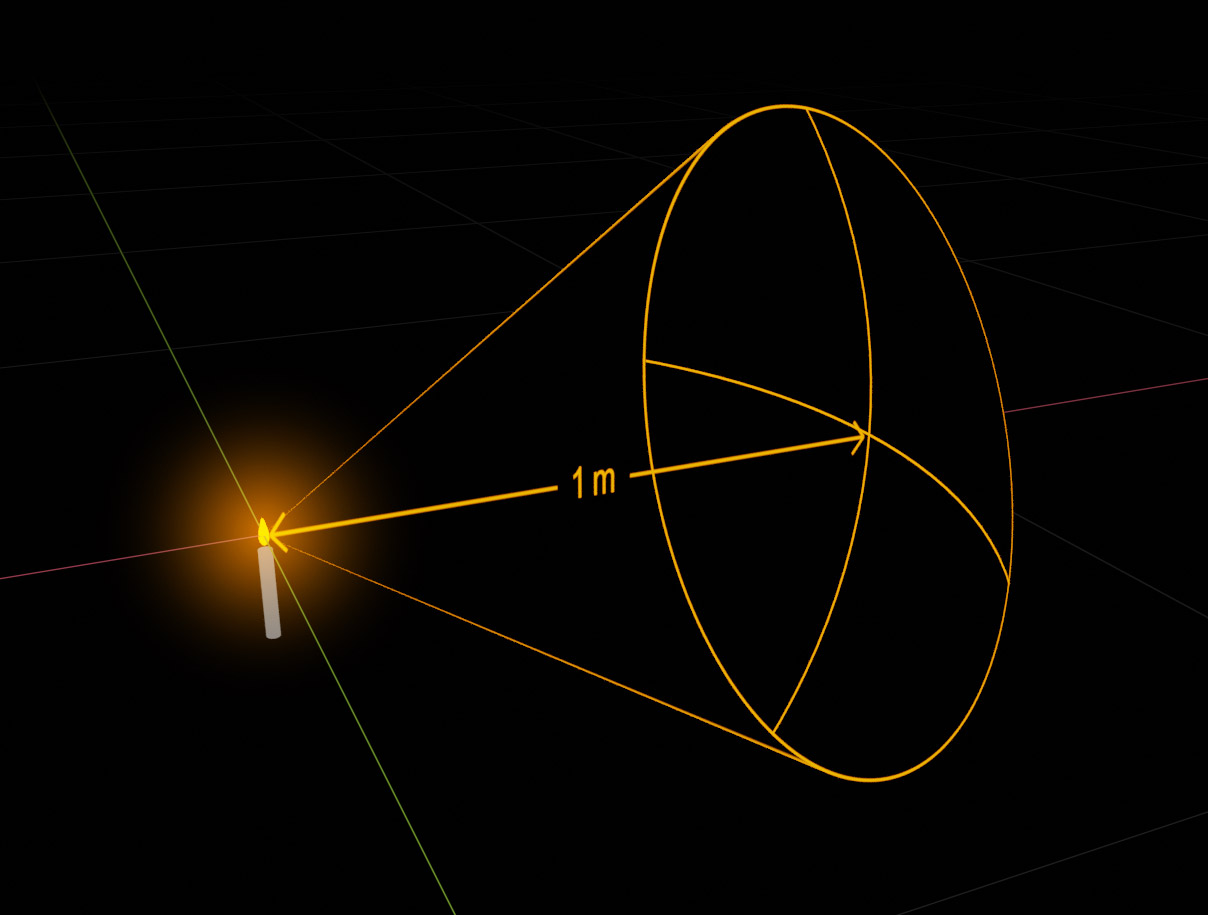## Understanding the Photometric Light Measurement Units

There are two sets of light intensity measurement units:
The Photometric units measure the intensity of visible light** as it is perceived by the human eye, and the Radiometric units measure the intensity of electromagnetic radiation***, which is the broader physical phenomenon of light, including the whole spectrum of radiation beyond visible light** (like x-rays and infrared radiation for example).

Light intensity is generally measured in three ways:

1. The directional intensity received from a light source as it is measured from a point in space. i.e Luminance in Photometric units or Radiance in Radiometric units.

2. The total light intensity output a light source emits to all directions i.e Luminous Flux in Photometric units or Radiant Flux in Radiometric units.

3. The amount of light intensity received by a surface from all directions i.e Illuminance in Photometric units or Irradiance in Radiometric units.

Similarly to the way measurement of kinetic power is based on the power of an ideal horse, the Photometric measurement units base the scale of light intensity on the light emitted by an ideal candle.Luminance (Candela):
When measured from any point in space, the Luminance of an ideal candle seen from that point is measured as 1Candela‘ i.e. 1CD‘.
> In 3D rendering, a photometric IES file describes a light source’s light beam pattern by listing the Luminance or CD of the light source in different directions.

For light emitting surfaces like LCD screens Luminance is measured as Candelas per 1 square meter of surface i.e. CD/m2. Typical LCD computer monitors for instance, have a Luminance of about 250 CD/m2. imagine your computer screen displaying pure white and extended to an area of 1m x 1m, the light intensity perceived from it would be as if about 250 candles were spread on the whole area.

Luminous Flux (Lumen):
The amount of light emitted by an ideal candle through 1 solid angleSteradian‘**** conic beam distribution is measured as 1Lumen‘ or 1lm‘. the total Luminous Flux of the candle in all directions is 4 x PI lumens i.e 12.56 lumens which is simply the whole surface area of the unit sphere.
> The total amount of light produced by different kind of light bulbs is usually specified by Luminous Flux measurement i.e how many Lumens does the light source output.
> If sn optical reflector is placed next to a light source, focusing all it’s light output to a narrow direction, it wont change the light source’s Luminous Flux (Lumen) output, but since the same Luminous Flux will be focused to a narrower beam, it will have a higher Luminance (CD) measured from that direction, and therefore surfaces at that direction will be receive a brighter Illumination (Lux) (see below).

Illuminance (Lux):
A 1 m2 (meter squared) area surface, receiving illumination of 1 lumen has a measured Illuminance of 1 lux or 1 lx. Illuminance is measured by how many lumens a surface receives per square meter.
> In photography, the amount of Illuminance at which a surface is lit is important for determining the proper photographic exposure for the picture.

The inverse-square law:
As a light beam travels through space it’s distribution covers a larger and larger area, therefore it’s energy per area is reduced. the light energy a candle emits through 1 solid angle steradian, in a distance of 1 meter will cover an area of 1 meter squared, therefore the area of 1 meter squared will receive 1 lumen of light energy and will be illuminated with an illuminance of 1 lux. as that 1 lumen of light energy travels another 1 meter further, to a distance of 2 meters from the candle, it spreads and covers an area of 4 meter squared. each square meter of the 4 now receives just 1/4 of a lumen, so it’s illuminated by only 1/4 lux. as that 1 lumen of light energy travels another 1 meter further, to a distance of 3 meters from the candle, it spreads and covers an area of 9 meter squared. each square meter of the 9 now receives just 1/9 of a lumen, so it’s illuminated by only 1/9 lux. after a distance of 4 meters, the same 1 lumen on light energy will be spread on an area of 16 meter squared, so each square meter will be illuminated by 1/16 lux. you can already see the emerging pattern, the illumination intensity is inversely proportional to the square of the distance to the light source. This phenomenon is referred to as ‘The inverse-square law‘, and in practical terms it means that surface illumination is greatly influenced by it’s distance from the light source.Notes:

* Radiometric units measure light intensity using Watt light energy units.
note that this isn’t the Watt measurement units of electric consumption we’re used to for classifying electric light sources with, but a Watt measurement of the actual energy in the light itself.

** Electromagnetic radiation of wave lengths that stimulate the human eye.

*** Also referred to as ‘light‘ in physics.

**** A ‘Steradian‘, also referred to as ‘square radian’ is a measurement unit of 3D conic span or ‘solid angle’. a solid angle of 1 Steradian beginning at the center of a unit sphere covers exactly an area of 1 squared on the surface of the sphere. (the whole surface area of the sphere being 4 PI). The Steradian can be thought of as the Radian’s 3 dimensional ‘cousin’.

Related posts:
IES lighting in CG
Fresnel reflections